# Floating point emulator

## Introduction

The Acorn RISC machine has a general coprocessor interface. The first coprocessor available is one which performs floating point calculations to the IEEE standard. To ensure that programs using floating point arithmetic remain compatible with all Archimedes machines, a standard ARM floating point instruction set has been defined. This can be implemented invisibly to the customer program by one of several systems offering various speed performances at various costs. The current 'bundled' floating point system is the software-only floating point emulator module. Floating point instructions may be incorporated into any assembler text, provided they are called from user mode. These instructions are recognised by the Assembler and converted into the correct coprocessor instructions. However, these instructions are not supported by the assembler in the BASIC interpreter.

Because this module doesn't present any SWIs or other usual interface to programs (apart from a SWI to return the version number), this chapter is structured differently from most others. First, there is a discussion of the programmer's model of the IEEE 754 floating point system. This is followed by the floating point instruction set. Finally the SWI is detailed.

Generally, programs do not need to know whether a coprocessor is fitted; the only effective difference is in the speed of execution. Note that there may be slight variations in accuracy between hardware and software - refer to the instructions supplied with the coprocessor for details of these variations.

## Programmer's model

The ARM IEEE floating point system has eight 'high precision' floating point registers, F0 to F7. The format in which numbers are stored in these registers is not specified. Floating point formats only become visible when a number is transferred to memory, using one of the formats described below.

There is also a floating point status register (FPSR) which, like the ARM's combined PC and PSR, holds all the necessary status and control information that an application is intended to be able to access. It holds flags which indicate various error conditions, such as overflow and division by zero. Each flag has a corresponding trap enable bit, which can be used to enable or disable a 'trap' associated with the error condition. Bits in the FPSR allow a client to distinguish between different implementations of the floating point system.

There may also be a floating point control register (FPCR); this is used to hold status and control information that an application is not intended to access. For example, there are privileged instructions to turn the floating point system on and off, to permit efficient context changes. Typically, hardware based systems have an FPCR, whereas software based ones do not.

#### Available systems

Floating point systems may be built from software only, hardware only, or some combination of software and hardware. The following terminology will be used to differentiate between the various ARM floating point systems already in use or planned:

System name System components
Old FPE Versions of the floating point emulator up to (but not including) 4.00
FPPC Floating Point Protocol Convertor (interface chip between ARM and WE32206), WE32206 (AT&T Math Acceleration Unit chip), and support code
FPE 400 Versions of the floating point emulator from 4.00 onwards
FPA ARM Floating Point Accelerator chip, and support code

The results look the same to the programmer. However, if clients are aware of which system is in use, they may be able to extract better performance.

The old FPE has two different variants. Versions up to (but not including) 3.40 do not provide any hardware support, whereas versions 3.40 to 3.99 inclusive provide support for the FPPC hardware - if it is fitted. All versions of the FPE 400 provide support for the FPA hardware.

#### Precision

All basic floating point instructions operate as though the result were computed to infinite precision and then rounded to the length, and in the way, specified by the instruction. The rounding is selectable from:

• Round to nearest
• Round to +infinity (P)
• Round to -infinity (M)
• Round to zero (Z).

The default is 'round to nearest'; in the event of a tie, this rounds to 'nearest even'. If any of the others are required they must be given in the instruction.

The working precision of the system is 80 bits, comprising a 64 bit mantissa, a 15 bit exponent and a sign bit. Specific instructions that work only with single precision operands may provide higher performance in some implementations, particularly the fully software based ones.

#### Floating point number formats

Like the ARM instructions, the floating point data processing operations refer to registers rather than memory locations. Values may be stored into ARM memory in one of five formats (only four of which are visible at any one time, since P and EP are mutually exclusive):

##### IEEE Single Precision (S)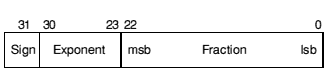Single precision format

• If the exponent is 0 and the fraction is 0, the number represented is ±0.
• If the exponent is 0 and the fraction is non-zero, the number represented is ±0.fraction × 2-126.
• If the exponent is in the range 1 to 254, the number represented is ±1.fraction × 2 exponent - 127.
• If the exponent is 255 and the fraction is 0, the number represented is ±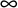.
• If the exponent is 255 and the fraction is non-zero, a NaN (not-a-number) is represented. If the most significant bit of the fraction is set, it is a non-trapping NaN; otherwise it is a trapping NaN.
##### IEEE Double Precision (D)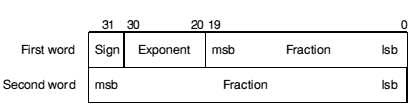Double precision format

• If the exponent is 0 and the fraction is 0, the number represented is ±0.
• If the exponent is 0 and the fraction is non-zero, the number represented is ±0.fraction × 2 -1022.
• If the exponent is in the range 1 to 2046, the number represented is ±1.fraction × 2 exponent - 1023.
• If the exponent is 2047 and the fraction is 0, the number represented is ±.
• If the exponent is 2047 and the fraction is non-zero, a NaN (not-a-number) is represented. If the most significant bit of the fraction is set, it is a non-trapping NaN; otherwise it is a trapping NaN.
##### Double Extended Precision (E)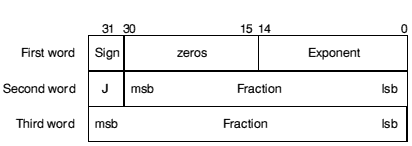Double extended precision format

• If the exponent is 0, J is 0, and the fraction is 0, the number represented is ±0.
• If the exponent is 0, J is 0, and the fraction is non-zero, the number represented is ±0.fraction × 2 -16382.
• If the exponent is in the range 0 to 32766, J is 1, and the fraction is non-zero, the number represented is ±1.fraction × 2 exponent - 16383.
• If the exponent is 32767, J is 0, and the fraction is 0, the number represented is ±.
• If the exponent is 32767 and the fraction is non-zero, a NaN (not-a-number) is represented. If the most significant bit of the fraction is set, it is a non-trapping NaN; otherwise it is a trapping NaN.

Other values are illegal and shall not be used (ie the exponent is in the range 1 to 32766 and J is 0; or the exponent is 32767, J is 1, and the fraction is 0).

The FPPC system stores the sign bit in bit 15 of the first word, rather than in bit 31.

Storing a floating point register in 'E' format is guaranteed to maintain precision when loaded back by the same floating point system in this format. Note that in the past the layout of E format has varied between floating point systems, so software should not have been written to depend on it being readable by other floating point systems. For example, no software should have been written which saves E format data to disc, to have then been potentially loaded into another system. In particular, E format in the FPPC system varies from all other systems in its positioning of the sign bit. However, for the FPA and the FPE 400, the E format is now defined to be a particular form of IEEE Double Extended Precision and will not vary in future.

##### Packed Decimal (P)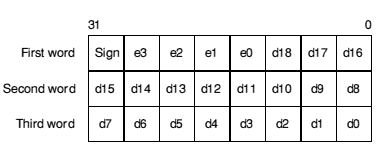Packed decimal format

The sign nibble contains both the significand's sign (top bit) and the exponent's sign (next bit); the other two bits are zero.

d18 is the most significant digit of the significand d, and e3 of the exponent e. The significand has an assumed decimal point between d18 and d17, and is normalised so that for a normal number lD189. The guaranteed ranges for d and e are 17 and 3 digits respectively; d0, d1 and e3 may always be zero in a particular system. (By comparison, an S format number has 9 digits of significand and a maximum exponent of 53; a D format number has 17 digits in the significand and a maximum exponent of 340.)

The result is undefined if any of the packed digits is hexadecimal A - F, save for a representation of ±or a NaN (see below).

• If the exponent's sign is 0, the exponent is 0, and the significand is 0, the number represented is ±0.
• If the exponent is in the range 0 to 9999 and the significand is in the range 1 to 9.999999999999999999, the number represented is ±d × 10 ±e .
• If the exponent is &FFFF (ie all the bits in e3 - e0 are set) and the significand is 0, the number represented is ±.
• If the exponent is &FFFF and d0 - d17 are non-zero, a NaN (not-a-number) is represented. If the most significant bit of d18 is set, it is a non-trapping NaN; otherwise it is a trapping NaN.

All other combinations are undefined.

##### Expanded Packed Decimal (EP)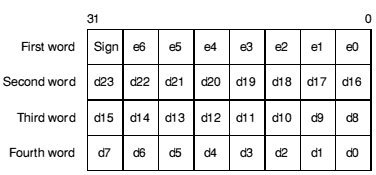Expanded packed decimal format

The sign nibble contains both the significand's sign (top bit) and the exponent's sign (next bit); the other two bits are zero.

d23 is the most significant digit of the significand d, and e6 of the exponent e. The significand has an assumed decimal point between d23 and d22, and is normalised so that for a normal number lD239. The guaranteed ranges for d and e are 21 and 4 digits respectively; d0, d1, d2, e4, e5 and e6 may always be zero in a particular system. (By comparison, an S format number has 9 digits of significand and a maximum exponent of 53; a D format number has 17 digits in the significand and a maximum exponent of 340.)

The result is undefined if any of the packed digits is hexadecimal A - F, save for a representation of ±or a NaN (see below).

• If the exponent's sign is 0, the exponent is 0, and the significand is 0, the number represented is ±0.
• If the exponent is in the range 0 to 9999999 and the significand is in the range 1 to 9.99999999999999999999999, the number represented is ±d × 10 ±e .
• If the exponent is &FFFFFFF (ie all the bits in e6 - e0 are set) and the significand is 0, the number represented is ±.
• If the exponent is &FFFFFFF and d0 - d22 are non-zero, a NaN (not-a-number) is represented. If the most significant bit of d23 is set, it is a non-trapping NaN; otherwise it is a trapping NaN.

All other combinations are undefined.

This format is not available in the old FPE or the FPPC. You should only use it if you can guarantee that the floating point system you are using supports it.

#### Floating point status register

There is a floating point status register (FPSR) which, like ARM's combined PC and PSR, has all the necessary status for the floating point system. The FPSR contains the IEEE flags but not the result flags - these are only available after floating point compare operations.

The FPSR consists of a system ID byte, an exception trap enable byte, a system control byte and a cumulative exception flags byte.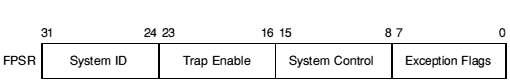Floating point status register byte usage

##### System ID byte

The System ID byte allows a user or operating system to distinguish which floating point system is in use. The top bit (bit 31 of the FPSR) is set for hardware (ie fast) systems, and clear for software (ie slow) systems. Note that the System ID is read-only.

The following System IDs are currently defined:

System System ID
Old FPE &00
FPPC &80
FPE 400 &01
FPA &81
##### Exception Trap Enable Byte

Each bit of the exception trap enable byte corresponds to one type of floating point exception, which are described in the section entitled Cumulative Exception Flags Byte.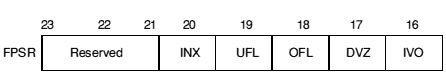Exception trap enable byte

If a bit in the cumulative exception flags byte is set as a result of executing a floating point instruction, and the corresponding bit is also set in the exception trap enable byte, then that exception trap will be taken.

Currently, the reserved bits shall be written as zeros and will return 0 when read.

##### System Control Byte

These control bits determine which features of the floating point system are in use.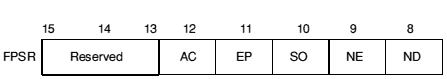System control byte

By placing these control bits in the FPSR, their state will be preserved across context switches, allowing different processes to use different features if necessary. The following five control bits are defined for the FPA system and the FPE 400:

 ND No Denormalised numbers NE NaN Exception SO Select synchronous Operation of FPA EP Use Expanded Packed decimal format AC Use Alternative definition for C flag on compare operations

The old FPE and the FPPC system behave as if all these bits are clear.

Currently, the reserved bits shall be written as zeros and will return 0 when read. Note that all bits (including bits 8 - 12) are reserved on FPPC and early FPE systems.

###### ND - No denormalised numbers bit

If this bit is set, then the software will force all denormalised numbers to zero to prevent lengthy execution times when dealing with denormalised numbers. (Also known as abrupt underflow or flush to zero.) This mode is not IEEE compatible but may be required by some programs for performance reasons.

If this bit is clear, then denormalised numbers will be handled in the normal IEEE-conformant way.

###### NE - NaN exception bit

If this bit is set, then an attempt to store a signalling NaN that involves a change of format will cause an exception (for full IEEE compatibility).

If this bit is clear, then an attempt to store a signalling NaN that involves a change of format will not cause an exception (for compatibility with programs designed to work with the old FPE).

###### SO - Select synchronous operation of FPA

If this bit is set, then all floating point instructions will execute synchronously and ARM will be made to busy-wait until the instruction has completed. This will allow the precise address of an instruction causing an exception to be reported, but at the expense of increased execution time.

If this bit is clear, then that class of floating point instructions that can execute asynchronously to ARM will do so. Exceptions that occur as a result of these instructions may be raised some time after the instruction has started, by which time the ARM may have executed a number of instructions following the one that has failed. In such cases the address of the instruction that caused the exception will be imprecise.

The state of this bit is ignored by software-only implementations, which always operate synchronously.

###### EP - Use expanded packed decimal format

If this bit is set, then the expanded (four word) format will be used for Packed Decimal numbers. Use of this expanded format allows conversion from extended precision to packed decimal and back again to be carried out without loss of accuracy.

If this bit is clear, then the standard (three word) format is used for Packed Decimal numbers.

###### AC - Use alternative definition for C flag on compare operations

If this bit is set, the ARM C flag, after a compare, is interpreted as 'Greater Than or Equal or Unordered'. This interpretation allows more of the IEEE predicates to be tested by means of single ARM conditional instructions than is possible using the original interpretation of the C flag (as shown below).

If this bit is clear, the ARM C flag, after a compare, is interpreted as 'Greater Than or Equal'.

##### Cumulative Exception Flags Byte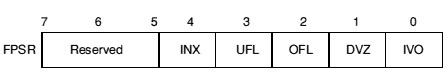Cumulative exception flags byte

Whenever an exception condition arises, the appropriate cumulative exception flag in bits 0 to 4 will be set to 1. If the relevant trap enable bit is set, then an exception is also delivered to the user's program in a manner specific to the operating system. (Note that in the case of underflow, the state of the trap enable bit determines under which conditions the underflow flag will be set.) These flags can only be cleared by a WFS instruction.

Currently, the reserved bits shall be written as zeros and will return 0 when read.

###### IVO - invalid operation

The IVO flag is set when an operand is invalid for the operation to be performed. Invalid operations are:

• Any operation on a trapping NaN (not-a-number)
• Magnitude subtraction of infinities, eg +P -• Multiplication of 0 by ±• Division of 0/0 or/• x REM y where x =or y = 0
• Square root of any number < 0 (but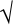(-0) = -0)
• Conversion to integer or decimal when overflow,or a NaN operand make it impossible
• Comparison with exceptions of Unordered operands
• ACS, ASN when argument's absolute value is > 1
• SIN, COS, TAN when argument is ±• LOG, LGN when argument is0
• POW when first operand is < 0 and second operand is not an integer, or first operand is 0 and second operand is0
• RPW when first operand is not an integer and second operand is < 0, or first operand is0 and second operand is 0.
###### DVZ - division by zero

The DVZ flag is set if the divisor is zero and the dividend a finite, non-zero number. A correctly signed infinity is returned if the trap is disabled.

The flag is also set for LOG(0) and for LGN(0). Negative infinity is returned if the trap is disabled.

###### OFL - overflow

The OFL flag is set whenever the destination format's largest number is exceeded in magnitude by what the rounded result would have been were the exponent range unbounded. As overflow is detected after rounding a result, whether overflow occurs or not after some operations depends on the rounding mode.

If the trap is disabled either a correctly signed infinity is returned, or the format's largest finite number. This depends on the rounding mode and floating point system used.

###### UFL - underflow

Two correlated events contribute to underflow:

• Tininess - the creation of a tiny non-zero result smaller in magnitude than the format's smallest normalised number.
• Loss of accuracy - a loss of accuracy due to denormalisation that may be greater than would be caused by rounding alone.

The UFL flag is set in different ways depending on the value of the UFL trap enable bit. If the trap is enabled, then the UFL flag is set when tininess is detected regardless of loss of accuracy. If the trap is disabled, then the UFL flag is set when both tininess and loss of accuracy are detected (in which case the INX flag is also set); otherwise a correctly signed zero is returned.

As underflow is detected after rounding a result, whether underflow occurs or not after some operations depends on the rounding mode.

###### INX - inexact

The INX flag is set if the rounded result of an operation is not exact (different from the value computable with infinite precision), or overflow has occurred while the OFL trap was disabled, or underflow has occurred while the UFL trap was disabled. OFL or UFL traps take precedence over INX.

The INX flag is also set when computing SIN or COS, with the exceptions of SIN(0) and COS(1).

The old FPE and the FPPC system may differ in their handling of the INX flag. Because of this inconsistency we recommend that you do not enable the INX trap.

#### Floating Point Control Register

The Floating Point Control register (FPCR) may only be present in some implementations: it is there to control the hardware in an implementation-specific manner, for example to disable the floating point system. The user mode of the ARM is not permitted to use this register (since the right is reserved to alter it between implementations) and the WFC and RFC instructions will trap if tried in user mode.

You are unlikely to need to access the FPCR; this information is principally given for completeness.

##### The FPPC system

The FPCR bit allocation in the FPPC system is as shown below: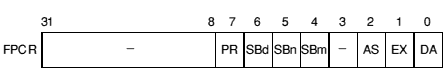FPCR bit allocation in the FPPC system

Bit Meaning
31-8 Reserved - always read as zero
7 PR Last RMF instruction produced a partial remainder
6 SBd Use Supervisor Register Bank 'd'
5 SBn Use Supervisor Register Bank 'n'
4 SBm Use Supervisor Register Bank 'm'
3 Reserved - always read as zero
2 AS Last WE32206 exception was asynchronous
1 EX Floating point exception has occurred
0 DA Disable

Reserved bits are ignored during write operations (but should be zero for future compatibility.) The reserved bits will return zero when read.

##### The FPA system

In the FPA, the FPCR will also be used to return status information required by the support code when an instruction is bounced. You should not alter the register unless you really know what you're doing. Note that the register will be read sensitive; even reading the register may change its value, with disastrous consequences.

The FPCR bit allocation in the FPA system is provisionally as follows:FPCR bit allocation in the FPA system

Bit Meaning
31 RU Rounded Up Bit
30 Reserved
29 Reserved
28 IE Inexact bit
27 MO Mantissa overflow
26 EO Exponent overflow
25, 24 Reserved
23-20 OP AU operation code
19 PR AU precision
18-16 S1 AU source register 1
15 OP AU operation code
14-12 DS AU destination register
11 SB Synchronous bounce: decode (R14) to get opcode
10 AB Asynchronous bounce: opcode supplied in rest of word
9 RE Rounding Exception: Asynchronous bounce occurred during rounding stage and destination register was written
8 EN Enable FPA (default is off)
7 PR AU precision
6, 5 RM AU rounding mode
4 OP AU operation code
3-0 S2 AU source register 2 (bit 3 set denotes a constant)

Note that the SB and AB bits are cleared on a read of the FPCR. Only the EN bit is writable. All other bits shall be set to zero on a write.

## The instruction set

#### Floating point coprocessor data transfer

 op is LDF for load, STF for store condition is one of the usual ARM conditions (see Appendix A: ARM assembler) prec is one of the usual floating point precisions (eg S for single, D for double, P for packed decimal: see the chapter entitled Floating point number formats) addr is [Rn]{,#offset} or [Rn,#offset]{!} ({!} if present indicates that writeback is to take place.) Fd is a floating point register symbol (defined via the FN directive).

Load (LDF) or store (STF) the high precision value from or to memory, using one of the five memory formats. On store, the value is rounded using the 'round to nearest' rounding method to the destination precision, or is precise if the destination has sufficient precision. Thus other rounding methods may be used by having previously applied some suitable floating point data operation; this does not compromise the requirement of 'rounding once only', since the store operation introduces no additional rounding error.

The offset is in words from the address given by the ARM base register, and is in the range -1020 to +1020. In pre-indexed mode you must explicitly specify writeback to add the offset to the base register; but in post-indexed mode the assembler forces writeback for you, as without write back post-indexing is meaningless.

You should not use R15 as the base register if writeback will take place.

Examples:

```LDFS    F0,[R0]         ; load F0 from address held in R0
; (single precision)
STFP    F1,[R2]         ; store number held in F1 at R2
; as a packed decimal number```

##### Floating point literals

LDFS and LDFD can be given literal values instead of a register relative address, and the Assembler will automatically place the required value in the next available literal pool. In the case of LDFS a single precision value is placed, in the case of LDFD a double precision value is placed. Because the allowed offset range within a LDFS or LDFD instruction is less than that for a LDR instruction (-1020 to +1020 instead of -4095 to +4095), it may be necessary to code LTORG directives more frequently if floating point literals are being used than would otherwise be necessary.

Syntax: LDFx Fn, = floating point number

#### Floating point coprocessor multiple data transfer

The LFM and SFM multiple data transfer instructions are supported by the assemblers, but are not provided by the FPPC system, or by some versions of the old FPE:

• versions 2.80 - 2.84 do not support them
• versions 2.85 - 3.39 do support them
• version 3.40 - which is effectively a version of 2.80 that also provides FPPC hardware support - does not support these instructions.

Attempting to execute these instructions on systems that do not provide them will cause undefined instruction traps, so you should only use these instructions in software intended for machines you are confident are using an appropriate version of the old FPE, or the FPE 400, or the FPA system.

The LFM and SFM instructions allow between 1 and 4 floating point registers to be transferred from or to memory in a single operation; such a transfer otherwise requires several LDF or STF operations. The multiple transfers are therefore useful for efficient stacking on procedure entry/exit and context switching. These new instructions are the preferred way to preserve exactly register contents within a program.

The values transferred to memory by SFM occupy three words for each register, but the data format used is not defined, and may vary between floating point systems. The only legal operation that can be performed on this data is to load it back into floating point registers using the LFM instruction. The data stored in memory by an SFM instruction should not be used or modified by any user process.

The registers transferred by a LFM or SFM instruction are specified by a base floating point register and the number of registers to be transferred. This means that a register set transferred has to have adjacent register numbers, unlike the unconstrained set of ARM registers that can be loaded or saved using LDM and STM. Floating point registers are transferred in ascending order, register numbers wrapping round from 7 to 0: eg transferring three registers with F6 as the base register results in registers F6, F7 then F0 being transferred.

The assembler supports two alternative forms of syntax, intended for general use or just stack manipulation:

op{condition}stacktype Fd,count,[Rn]{!}

 op is LFM for load, SFM for store. condition is one of the usual ARM conditions. Fd is the base floating point register, specified as a floating point register symbol (defined via the FN directive). count is an integer from 1 to 4 specifying the number of registers to be transferred. addr is [Rn]{,#offset} or [Rn,#offset]{!} ({!} if present indicates that writeback is to take place). stacktype is FD or EA, standing for Full Descending or Empty Ascending, the meanings as for LDM and STM.

The offset (only relevant for the first, general, syntax above) is in words from the address given by the ARM base register, and is in the range -1020 to +1020. In pre-indexed mode you must explicitly specify writeback to add the offset to the base register; but in post-indexed mode the assembler forces writeback for you, as without write back post-indexing is meaningless.

You should not use R15 as the base register if writeback will take place.

Examples:

```SFMNE   F6,4,[R0]       ;if NE is true, transfer F6, F7,
;F0 and F1 to the address
;contained in R0
LFMFD   F4,2,[R13]!     ;load F4 and F5 from FD stack -
LFM     F4,2,[R13],#24  ;equivalent to same instruction
;in general syntax```

#### Floating point coprocessor register transfer

 FLT{condition}prec{round} Fn,Rd FLT{condition}prec{round} Fn,#value FIX{condition}{round} Rd,Fn WFS{condition} Rd RFS{condition} Rd WFC{condition} Rd RFC{condition} Rd
 {round} is the optional rounding mode: P, M or Z; see below. Rd is an ARM register symbol. Fn is a floating point register symbol.

The value may be of the following: 0, 1, 2, 3, 4, 5, 10, 0.5. Note that these values must be written precisely as shown above, for instance '0.5' is correct but '.5' is not.

 FLT Integer to Floating Point Fn := Rd FIX Floating point to integer Rd := Fm WFS Write Floating Point Status FPSR := Rd RFS Read Floating Point Status Rd := FPSR WFC Write Floating Point Control FPC := R Supervisor Only RFC Read Floating Point Control Rd := FPC Supervisor Only

The rounding modes are:

Mode Letter
Nearest (no letter required)
Plus infinity P
Minus infinity M
Zero Z

#### Floating point coprocessor data operations

The formats of these instructions are:

binop{condition}prec{round} Fd, Fn, Fm

binop{condition}prec{round} Fd, Fn, #value

unop{condition}prec{round} Fd, Fm

unop{condition}prec{round} Fd, #value

 binop is one of the binary operations listed below unop is one of the unary operations listed below Fd is the FPU destination register Fn is the FPU source register (binops only) Fm is the FPU source register #value is a constant, as an alternative to Fm. It must be 0, 1, 2, 3, 4, 5, 10 or 0.5, as above.

The binops are:

 ADF Add Fd := Fn + Fm MUF Multiply Fd := Fn × Fm SUF Subtract Fd := Fn - Fm RSF Reverse Subtract Fd := Fm - Fn DVF Divide Fd := Fn/Fm RDF Reverse Divide Fd := Fm/Fn POW Power Fd := Fn to the power of Fm RPW Reverse Power Fd := Fm to the power of Fn RMF Remainder Fd := remainder of Fn / Fm (Fd := Fn - integer value of (Fn/Fm) × Fm) FML Fast Multiply Fd := Fn × Fm FDV Fast Divide Fd := Fn / Fm FRD Fast Reverse Divide Fd := Fm / Fn POL Polar angle Fd := polar angle of Fn, Fm

The unops are:

 MVF Move Fd := Fm MNF Move Negated Fd := -Fm ABS Absolute value Fd := ABS (Fm) RND Round to integral value Fd := integer value of Fm SQT Square root Fd := square root of Fm LOG Logarithm to base 10 Fd := log Fm LGN Logarithm to base e Fd := ln Fm EXP Exponent Fd := e to the power of Fm SIN Sine Fd := sine of Fm COS Cosine Fd := cosine of Fm TAN Tangent Fd := tangent of Fm ASN Arc Sine Fd := arcsine of Fm ACS Arc Cosine Fd := arccosine of Fm ATN Arc Tangent Fd := arctangent of Fm URD Unnormalised Round Fd := integer value of Fm (may be abnormal) NRM Normalise Fd := normalised form of Fm

Note that wherever Fm is mentioned, one of the floating point constants 0, 1, 2, 3, 4, 5, 10, or 0.5 can be used instead.

FML, FRD and FDV are only defined to work with single precision operands. These 'fast' instructions are likely to be faster than the equivalent MUF, DVF and RDF instructions, but this is not necessarily so for any particular implementation.

Rounding is done only at the last stage of a SIN, COS etc - the calculations to compute the value are done with 'round to nearest' using the full working precision.

The URD and NRM operations are only supported by the FPA and the FPE 400.

#### Floating point coprocessor status transfer

op{condition}prec{round} Fm, Fn

op is one of the following:

 CMF Compare floating compare Fn with Fm CNF Compare negated floating compare Fn with -Fm CMFE Compare floating with exception compare Fn with Fm CNFE Compare negated floating with exception compare Fn with -Fm
 {condition} an ARM condition prec a precision letter {round} an optional rounding mode: P, M or Z Fm A floating point register symbol. Fn A floating point register symbol.

Compares are provided with and without the exception that could arise if the numbers are unordered (ie one or both of them is not-a-number). To comply with IEEE 754, the CMF instruction should be used to test for equality (ie when a BEQ or BNE is used afterwards) or to test for unorderedness (in the V flag). The CMFE instruction should be used for all other tests (BGT, BGE, BLT, BLE afterwards).

When the AC bit in the FPSR is clear, the ARM flags N, Z, C, V refer to the following after compares:

 N Less than ie Fn less than Fm (or -Fm) Z Equal C Greater than or equal ie Fn greater than or equal to Fm (or -Fm) V Unordered

Note that when two numbers are not equal, N and C are not necessarily opposites. If the result is unordered they will both be clear.

When the AC bit in the FPSR is set, the ARM flags N, Z, C, V refer to the following after compares:

 N Less than Z Equal C Greater than or equal or unordered V Unordered

In this case, N and C are necessarily opposites.

#### Finding out more...

Further details of the floating point instructions (such as the format of the bitfields within the instruction) can be found in the Acorn RISC Machine family Data Manual. VLSI Technology Inc. (1990) Prentice-Hall, Englewood Cliffs, NJ, USA: ISBN 0-13-781618-9 and in the Acorn Assembler Release 2 manual.

## FPEmulator_Version(SWI &40480)

Returns the version number of the floating point emulator

--

##### On exit

R0 = BCD version number

##### Interrupts

Interrupt status is undefined
Fast interrupts are enabled

##### Processor mode

Processor is in SVC mode

Not defined

##### Use

This call returns the version number of the floating point emulator as a binary coded decimal (BCD) number in R0.

This SWI will continue to be supported by the hardware expansion.

None

None